# Statistics

Statistics is the study of the collection, analysis, interpretation, presentation, and organization of data. In other words, it is a mathematical discipline to collect, summarize data. Also, we can say that statistics is a branch of applied mathematics. However, there are two important and basic ideas involved in statistics; they are uncertainty and variation. The uncertainty and variation in different fields can be determined only through statistical analysis. These uncertainties are basically determined by the probability that plays an important role in statistics.

## What is Statistics?

Statistics is simply defined as the study and manipulation of data. As we have already discussed in the introduction that statistics deals with the analysis and computation of numerical data. Let us see more definitions of statistics given by different authors here.

According to Merriam-Webster dictionary, statistics is defined as “classified facts representing the conditions of a people in a state – especially the facts that can be stated in numbers or any other tabular or classified arrangement”.

According to statistician Sir Arthur Lyon Bowley, statistics is defined as “Numerical statements of facts in any department of inquiry placed in relation to each other”.

Download the PDF to get the statistics notes and learn offline too.

## Statistics Examples

Some of the real-life examples of statistics are:

• To find the mean of the marks obtained by each student in the class whose strength is 50. The average value here is the statistics of the marks obtained.
• Suppose you need to find how many members are employed in a city. Since the city is populated with 15 lakh people, hence we will take a survey here for 1000 people (sample). Based on that, we will create the data, which is the statistic.

## Basics of Statistics

The basics of statistics include the measure of central tendency and the measure of dispersion. The central tendencies are mean, median and mode and dispersions comprise variance and standard deviation.

Mean is the average of the observations. Median is the central value when observations are arranged in order. The mode determines the most frequent observations in a data set.

Variation is the measure of spread out of the collection of data. Standard deviation is the measure of the dispersion of data from the mean. The square of standard deviation is equal to the variance.

## Mathematical Statistics

Mathematical statistics is the application of Mathematics to Statistics, which was initially conceived as the science of the state — the collection and analysis of facts about a country: its economy, and, military, population, and so forth.

Mathematical techniques used for different analytics include mathematical analysis, linear algebra, stochastic analysis, differential equation and measure-theoretic probability theory.

## Types of Statistics

Basically, there are two types of statistics.

• Descriptive Statistics
• Inferential Statistics

In the case of descriptive statistics, the data or collection of data is described in summary. But in the case of inferential stats, it is used to explain the descriptive one. Both these types have been used on large scale.

Descriptive Statistics

The data is summarised and explained in descriptive statistics. The summarization is done from a population sample utilising several factors such as mean and standard deviation. Descriptive statistics is a way of organising, representing, and explaining a set of data using charts, graphs, and summary measures. Histograms, pie charts, bars, and scatter plots are common ways to summarise data and present it in tables or graphs. Descriptive statistics are just that: descriptive. They don’t need to be normalised beyond the data they collect.

Inferential Statistics

We attempt to interpret the meaning of descriptive statistics using inferential statistics. We utilise inferential statistics to convey the meaning of the collected data after it has been collected, evaluated, and summarised. The probability principle is used in inferential statistics to determine if patterns found in a study sample may be extrapolated to the wider population from which the sample was drawn. Inferential statistics are used to test hypotheses and study correlations between variables, and they can also be used to predict population sizes. Inferential statistics are used to derive conclusions and inferences from samples, i.e. to create accurate generalisations.

## Statistics Formulas

The formulas that are commonly used in statistical analysis are given in the table below.

 Sample Mean ($$\begin{array}{l}\bar{x}\end{array}$$) $$\begin{array}{l}\frac{\sum x}{n}\end{array}$$ Population Mean ($$\begin{array}{l}\mu\end{array}$$) $$\begin{array}{l}\frac{\sum x}{N}\end{array}$$ Sample Standard Deviation (s) $$\begin{array}{l}\sqrt{\frac{\sum (x-\bar{x})^{2} }{n-1}}\end{array}$$ Population Standard Deviation ($$\begin{array}{l}\sigma\end{array}$$) $$\begin{array}{l}\sigma = \sqrt{\frac{(x-\mu )^{2}}{N}}\end{array}$$ Sample Variance ($$\begin{array}{l}s^{2}\end{array}$$) $$\begin{array}{l}s^{2} = \frac{\sum (x_{i}-\bar{x})^{2}}{n-1}\end{array}$$ Population Variance ($$\begin{array}{l}\sigma ^{2}\end{array}$$) $$\begin{array}{l}\sigma ^{2} = \frac{\sum (x_{i} – \mu)^{2}}{N}\end{array}$$ Range (R) Largest data value – smallest data value

### Summary Statistics

In Statistics, summary statistics are a part of descriptive statistics (Which is one of the types of statistics), which gives the list of information about sample data. We know that statistics deals with the presentation of data visually and quantitatively. Thus, summary statistics deals with summarizing the statistical information. Summary statistics generally deal with condensing the data in a simpler form, so that the observer can understand the information at a glance. Generally, statisticians try to describe the observations by finding:

• The measure of central tendency or mean of the locations, such as arithmetic mean.
• The measure of distribution shapes like skewness or kurtosis.
• The measure of dispersion such as the standard mean absolute deviation.
• The measure of statistical dependence such as correlation coefficient.

Summary Statistics Table

The summary statistics table is the visual representation of summarized statistical information about the data in tabular form.

For example, the blood group of 20 students in the class are O, A, B, AB, B, B, AB, O, A, B, B, AB, AB, O, O, B, A, AB, B, A.

 Blood Group No. of Students O 4 A 4 B 7 AB 5 Total 20

Thus, the summary statistics table shows that 4 students in the class have O blood group, 4 students have A blood group, 7 students in the class have B blood group and 5 students in the class have AB blood group. The summary statistics table is generally used to represent the big data related to population, unemployment, and the economy to be summarized systematically to interpret the accurate result.

## Scope of Statistics

Statistics is used in many sectors such as psychology, geology, sociology, weather forecasting, probability and much more. The goal of statistics is to gain understanding from the data, it focuses on applications, and hence, it is distinctively considered as a mathematical science.

## Methods in Statistics

The methods involve collecting, summarizing, analyzing, and interpreting variable numerical data. Here some of the methods are provided below.

• Data collection
• Data summarization
• Statistical analysis

## What is Data in Statistics?

Data is a collection of facts, such as numbers, words, measurements, observations etc.

### Types of Data

1. Qualitative data- it is descriptive data.
• Example- She can run fast, He is thin.
2. Quantitative data- it is numerical information.
• Example- An Octopus is an Eight legged creature.

### Types of quantitative data

1. Discrete data- has a particular fixed value. It can be counted
2. Continuous data- is not fixed but has a range of data. It can be measured.

### Representation of Data

There are different ways to represent data such as through graphs, charts or tables. The general representation of statistical data are:

• Bar Graph
• Pie Chart
• Line Graph
• Pictograph
• Histogram
• Frequency Distribution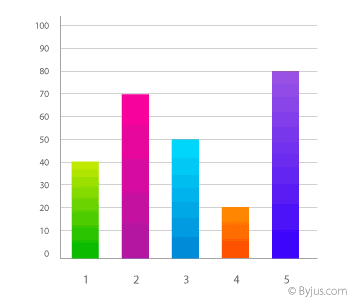Bar Graph A Bar Graph represents grouped data with rectangular bars with lengths proportional to the values that they represent. The bars can be plotted vertically or horizontally.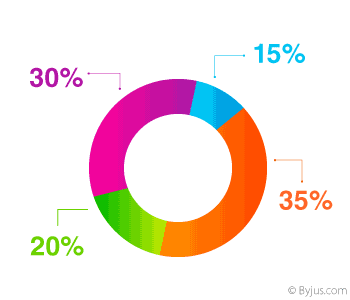Pie Chart A type of graph in which a circle is divided into Sectors. Each of these sectors represents a proportion of the whole.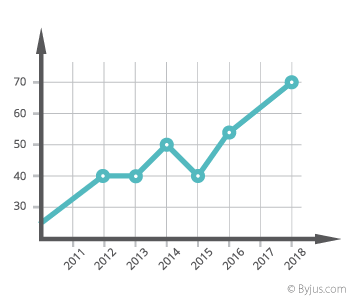Line graph The line chart is represented by a series of data points connected with a straight line. The series of data points are called ‘markers.’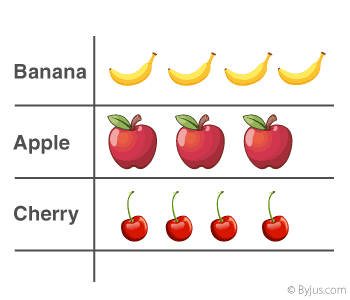Pictograph A pictorial symbol for a word or phrase, i.e. showing data with the help of pictures. Such as Apple, Banana & Cherry can have different numbers, and it is just a representation of data.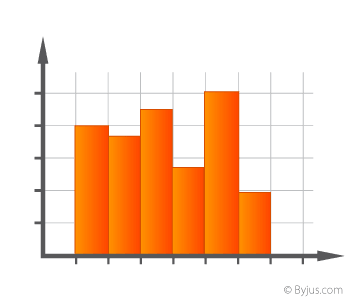Histogram A diagram is consisting of rectangles. Whose area is proportional to the frequency of a variable and whose width is equal to the class interval.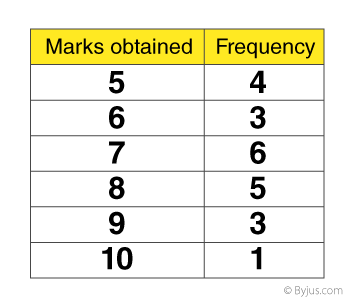Frequency Distribution The frequency of a data value is often represented by “f.” A frequency table is constructed by arranging collected data values in ascending order of magnitude with their corresponding frequencies.

## Measures of Central Tendency

In Mathematics, statistics are used to describe the central tendencies of the grouped and ungrouped data. The three measures of central tendency are:

All three measures of central tendency are used to find the central value of the set of data.

## Measures of Dispersion

In statistics, the dispersion measures help interpret data variability, i.e. to understand how homogenous or heterogeneous the data is. In simple words, it indicates how squeezed or scattered the variable is. However, there are two types of dispersion measures, absolute and relative. They are tabulated as below:

 Absolute measures of dispersion Relative measures of dispersion Co-efficient of Range Co-efficient of Variation Co-efficient of Standard Deviation Co-efficient of Quartile Deviation Co-efficient of Mean Deviation

## Skewness in Statistics

Skewness, in statistics, is a measure of the asymmetry in a probability distribution. It measures the deviation of the curve of the normal distribution for a given set of data.

The value of skewed distribution could be positive or negative or zero. Usually, the bell curve of normal distribution has zero skewness.

## ANOVA Statistics

ANOVA Stands for Analysis of Variance. It is a collection of statistical models, used to measure the mean difference for the given set of data.

## Degrees of freedom

In statistical analysis, the degree of freedom is used for the values that are free to change. The independent data or information that can be moved while estimating a parameter is the degree of freedom of information.

## Applications of Statistics

Statistics have huge applications across various fields in Mathematics as well as in real life. Some of the applications of statistics are given below:

• Applied statistics, theoretical statistics and mathematical statistics
• Machine learning and data mining
• Statistics in society
• Statistical computing
• Statistics applied to the mathematics of the arts

## Video Lesson## Statistics Related Articles

Hope this detailed discussion and formulas on statistics will help you to solve problems quickly and efficiently. Learn more Maths concepts at BYJU’S with the help of interactive videos.

## Frequently Asked Questions on Statistics

### What exactly is statistics?

Statistics is a branch that deals with the study of the collection, analysis, interpretation, organisation, and presentation of data. Mathematically, statistics is defined as the set of equations, which are used to analyse things.

### What are the two types of statistics?

The two different types of statistics used for analyzing the data are:

• Descriptive Statistics: It summarizes the data from the sample using indexes
• Inferential Statistics: It concludes from the data which are subjected to the random variation

### What is Summary Statistics?

Summary statistics is a type of descriptive statistics, which is used to summarize the set of observations with large information as simply as possible. Statisticians used to describe the observation by finding the measures of central tendency, statistical dispersion, statistical dependence, and the shape of the distribution.

### How is statistics applicable in Maths?

Statistics is a part of Applied Mathematics that uses probability theory to generalize the collected sample data. It helps to characterize the likelihood where the generalizations of data are accurate. This is known as statistical inference.

### What is the purpose of statistics?

Statistics make us learn to utilize a restricted sample to make accurate determinations about a more prominent populace. The utilization of tables, diagrams, and graphs assumes a crucial part in introducing the information being utilized to reach these determinations.

### What is the importance of Statistics in real life?

Statistics encourages you to utilize legitimate strategies to gather the information, utilize the right examinations, and successfully present the outcomes. Measurement is a significant cycle behind how we make disclosures in science, settle on choices dependent on information, and make forecasts.